# 模式搜索的暴力方法（第二部分）：深入

### 暴力程序的新版本和更改列表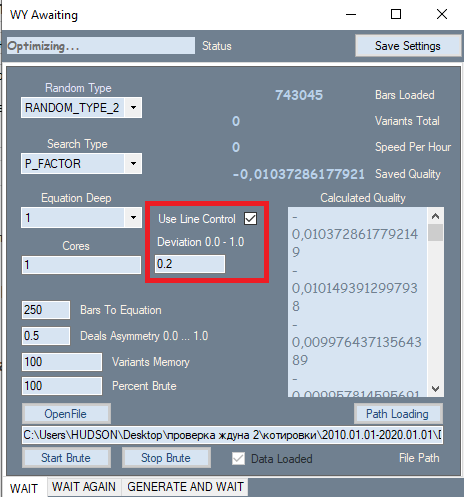• Close[i]-Open[i]
• High[i]-Open[i]
• Low[i]-Close[i]
• Low[i]-Open[i]
• High[i]-Close[i]

• C1*(High[i]-Close[i]) + C2*(Low[i]-Close[i]) = NewQuant
• if  C1 = 1 and C2 = -1
• NewQuant = (High[i]-Close[i]) - (Low[i]-Close[i]) =  High[i]-Low[i]
• 其它情况下
• NewQuant = (C1-C3)*(High[i]-Close[i])+(C2+C3)*(Low[i]-Close[i])  +  C3*((High[i]-Close[i]) - (Low[i]-Close[i])) = (C1-C3)*(High[i]-Close[i])+(C2-C3)*(Low[i]-Close[i]) +  C3*(High[i]-Low[i])

• Y = Sum(0,NC-1)( C[i]*Variant of product(x^p*x^p...*x[N]^p[N]) )
• Sum(0,N)(p[i])=MaxPowOfPolinom
• NC - 多项式中项的总数，等于所选系数的个数

1. x[i] =Close[i]-Open[i]
2. x[i] =High[i]-Open[i]
3. x[i] =Low[i]-Close[i]
4. x[i] =High[i]-Open[i]
5. x[i] =High[i]-Close[i]

• 优化函数的唯一性
• 信号强度与函数值的关系
• 易用性
• 唯一可优化的参数是多项式值

• Mn(t) = W(t,F)*Ef(T,N,L)
• Mn -  数学上期望的满足我们要求的策略数目
• W(t,F) = F*t - 每单位时间内检查的变体的结果数
• Ef(T,N,L) = ? - 暴力效率，取决于间隔长度、报价类型、所需的关闭订单数和所需的线性系数（如果在暴力期间考虑到这一点）（该值等于当前变量的结果采用必要和充分值的概率）
• N - 我们测试所允许的最小交易数量
• L - 最大允许线性系数（作为附加控制标准）
• F - 策略变量暴力频率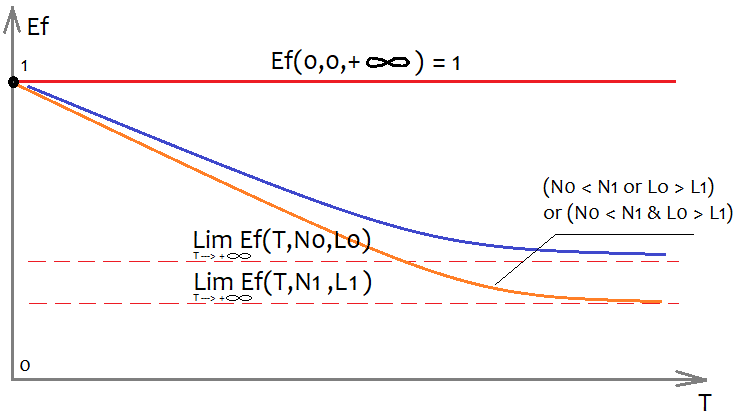• Mq=Mq(T,N,S)
• S - 选定的公式配置

• P_FACTOR=(Profit-Loss)/(Profit+Loss)

• Mx=Mx(T)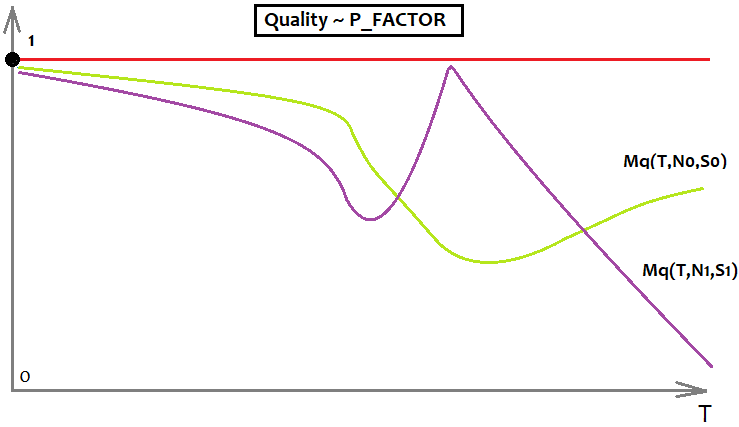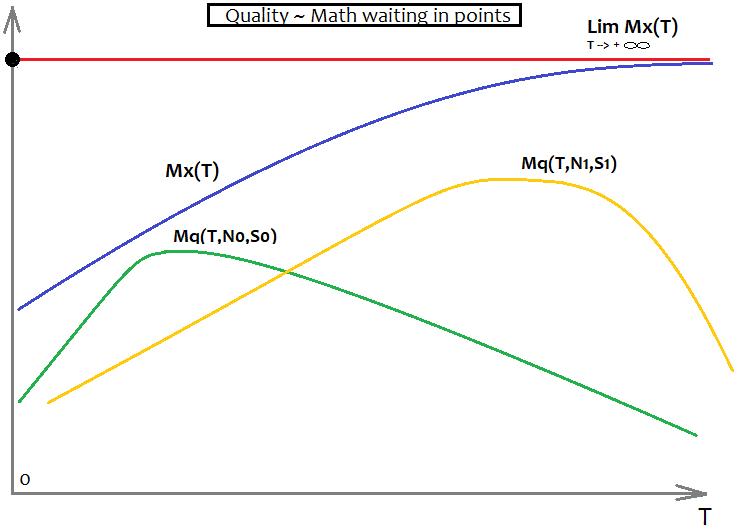• 几乎所有的量都不是固定的，而是由它们的数学期望所代替。
• 大样本的数学期望值倾向于全局样本的结果
• 更频繁地使用概率

### 模板变更

1. 我们可以控制所有可能的因素排列
2. 用总系数除以公式中重复因子的个数，以补偿公式中重复因子的个数

• Comb=n!/(n-k)!
• n 是可能的独立乘数的数目
• k 是最后的总次数

```double Combinations(int k,int n)
{
int i1=1;
int i2=1;
for ( int i=n; i<n-k; i-- ) i1*=i;
for ( int i=n-k; i>1; i-- ) i2*=i;
return double(i1/i2);
}
```

```double Val;
int iterator;
{
Val=0;
iterator=0;
if ( DeepBruteX <= 1 )
{
for ( int i=0; i<CNum; i++ )
{
Val+=C1[iterator]*(Close[i+1]-Open[i+1])/Point;
iterator++;
}

for ( int i=0; i<CNum; i++ )
{
Val+=C1[iterator]*(High[i+1]-Open[i+1])/Point;
iterator++;
}

for ( int i=0; i<CNum; i++ )
{
Val+=C1[iterator]*(Open[i+1]-Low[i+1])/Point;
iterator++;
}

for ( int i=0; i<CNum; i++ )
{
Val+=C1[iterator]*(High[i+1]-Close[i+1])/Point;
iterator++;
}

for ( int i=0; i<CNum; i++ )
{
Val+=C1[iterator]*(Close[i+1]-Low[i+1])/Point;
iterator++;
}

return Val;
}
else
{
CalcDeep(C1,CNum,DeepBruteX);
return ValStart;
}
}

///Fractal calculation of numbers
double ValW;//the number where everything is multiplied (and then added to ValStart)
uint NumC;//the current number for the coefficient
double ValStart;//the number where to add everything
void Deep(double &Ci0[],int Nums,int deepC,int deepStart,double Val0=1.0)//intermediary fractal
{
for ( int i=0; i<Nums; i++ )
{
if (deepC > 1)
{
ValW=(Close[i+1]-Open[i+1])*Val0;
Deep(Ci0,Nums,deepC-1,deepStart,ValW);
}
else
{
ValStart+=(Ci0[NumC]/Combinations(deepStart,Nums*5))*(Close[i+1]-Open[i+1])*Val0/Point;
NumC++;
}
}

for ( int i=0; i<Nums; i++ )
{
if (deepC > 1)
{
ValW=(High[i+1]-Open[i+1])*Val0;
Deep(Ci0,Nums,deepC-1,deepStart,ValW);
}
else
{
ValStart+=(Ci0[NumC]/Combinations(deepStart,Nums*5))*(High[i+1]-Open[i+1])*Val0/Point;
NumC++;
}
}

for ( int i=0; i<Nums; i++ )
{
if (deepC > 1)
{
ValW=(Open[i+1]-Low[i+1])*Val0;
Deep(Ci0,Nums,deepC-1,deepStart,ValW);
}
else
{
ValStart+=(Ci0[NumC]/Combinations(deepStart,Nums*5))*(Open[i+1]-Low[i+1])*Val0/Point;
NumC++;
}
}

for ( int i=0; i<Nums; i++ )
{
if (deepC > 1)
{
ValW=(High[i+1]-Close[i+1])*Val0;
Deep(Ci0,Nums,deepC-1,deepStart,ValW);
}
else
{
ValStart+=(Ci0[NumC]/Combinations(deepStart,Nums*5))*(High[i+1]-Close[i+1])*Val0/Point;
NumC++;
}
}

for ( int i=0; i<Nums; i++ )
{
if (deepC > 1)
{
ValW=(Close[i+1]-Low[i+1])*Val0;
Deep(Ci0,Nums,deepC-1,deepStart,ValW);
}
else
{
ValStart+=(Ci0[NumC]/Combinations(deepStart,Nums*5))*(Close[i+1]-Low[i+1])*Val0/Point;
NumC++;
}
}
}

void CalcDeep(double &Ci0[],int Nums,int deepC=1)
{
NumC=0;
ValStart=0.0;
for ( int i=0; i<deepC; i++ ) Deep(Ci0,Nums,i+1,i+1);
}
```

```int NumCAll=0;//size of the array of coefficients
void DeepN(int Nums,int deepC=1)//intermediate fractal
{
for ( int i=0; i<Nums*5; i++ )
{
if (deepC > 1) DeepN(Nums,deepC-1);
else NumCAll++;
}
}

void CalcDeepN(int Nums,int deepC=1)
{
NumCAll=0;
for ( int i=0; i<deepC; i++ ) DeepN(Nums,i+1);
}
```

### 分析找到的模式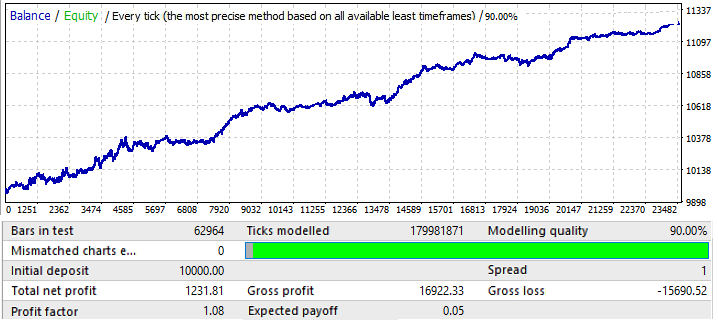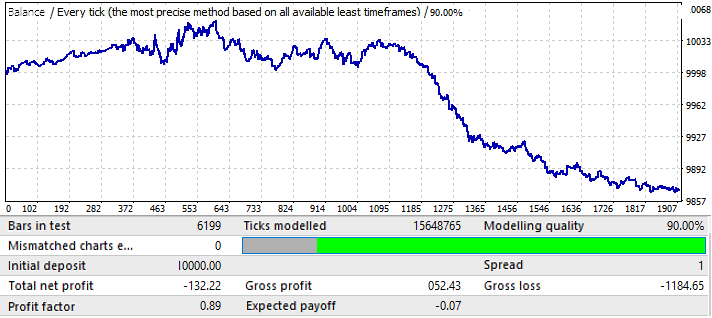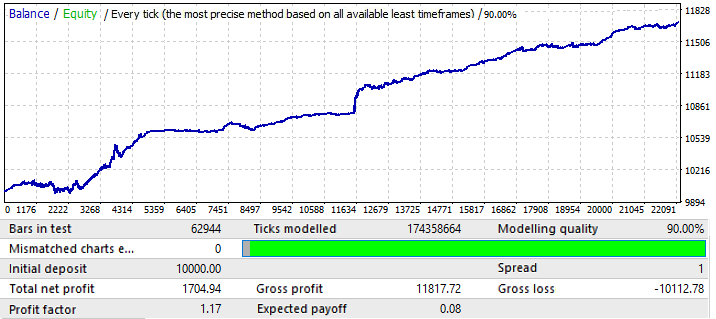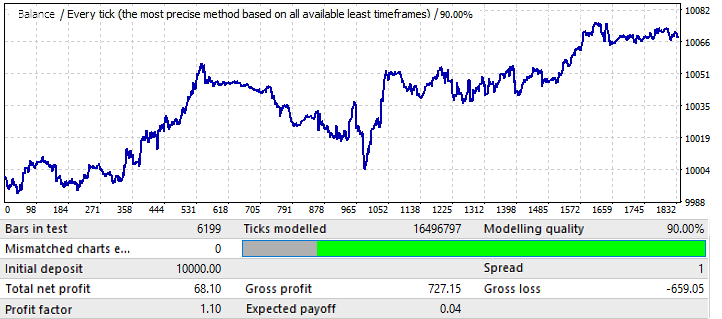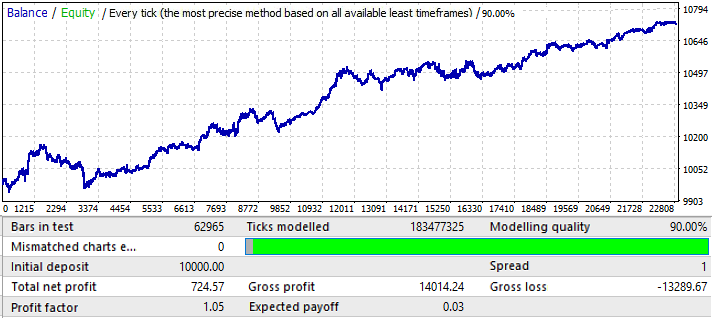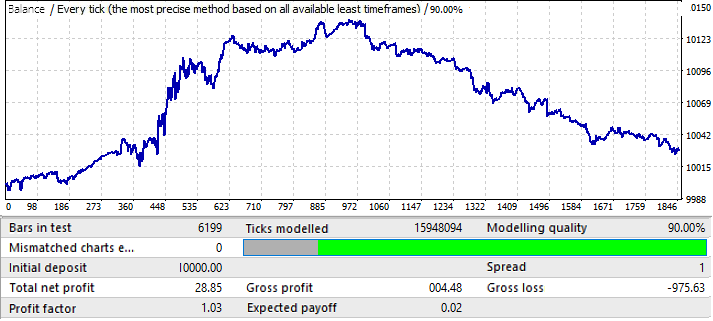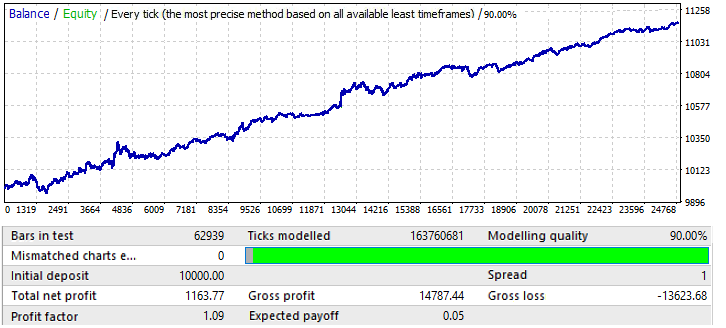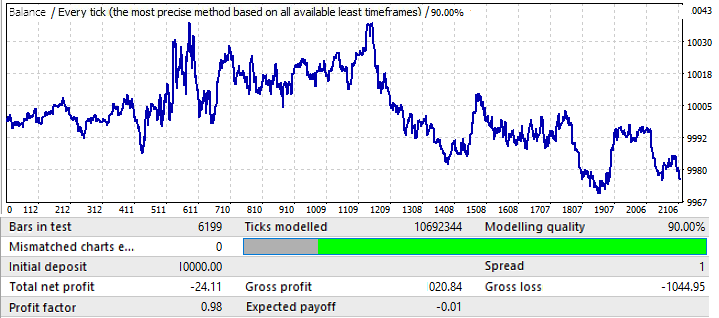1. 显然，EURCHF 是最好的图表
2. 无论是在训练期还是在前向测试中，EURCHF 都优于其他所有的货币对
3. 在除 EURCHF 以外的所有图表中，这一模式一直保持到年中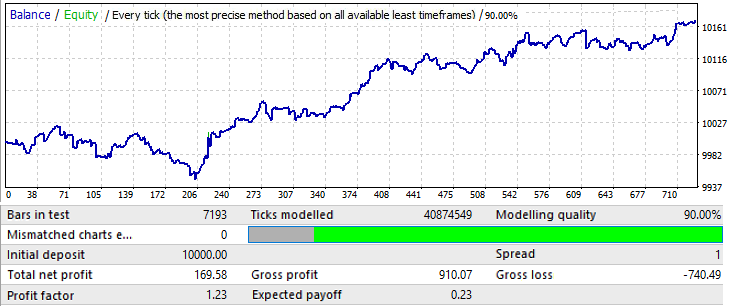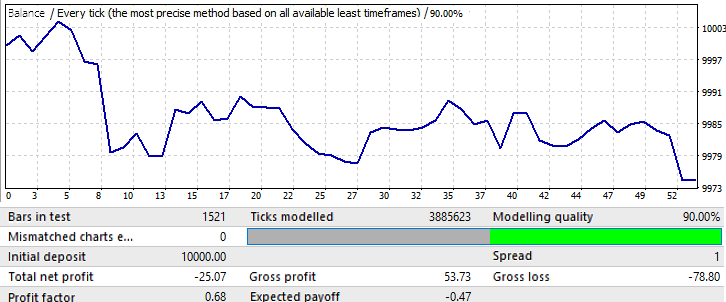### 数据分析

• EA交易，利用全局模式，并能够在相当长的时间内运作，可以创建一个简单的数字暴力算法
• 未来有效的持续时间直接取决于数据样本的大小
• 有效运行时间的长短直接取决于训练区的测试质量
• 在进行远期测试时，所有图表上全局模式的有效性至少保持2-3个月
• 当暴力计算部分最终结果的质量趋于100%时，找到一个至少还能工作几年的模式的可能性就会增加
• 当我们搜索全局模式时，不同的设置对不同的货币对起着不同的作用

• 模式一直在改变
• 可以小心地使用马丁格尔

1. 利用全局模式
2. 利用局部模式

• 未来“F”柱有无数可能的发展（无论是否有订单打开）
• 对于未来开发的所有选项，其中订单在特定柱上打开，此订单将具有以点数和利润系数表示的预期收益（假设您在一个柱上进行测试，对于每个订单，只有以下柱始终不同）
• 每个公式的所有这些量都是不同的
• 对于具有不同未来开始日期（未来的开始是训练部分的结束）和不同训练部分持续时间（相当于训练部分的不同开始日期）的部分，最终寻求的参数将采用唯一的值

• MF=MF(T,I,F,S,R) — 未来特定柱上订单的数学期望。
• PF=PF(T,I,F,S,R)  — 未来特定柱上订单的利润因子。
• T  — 训练部分的持续时间（在这种情况下是样本大小或柱数）。
• I  — 训练部分结束的日期。
• F  — 未来柱的编号，从训练结束日期开始。
• S  — 一个唯一的多项式或一个算法（如果模式是手动或由另一个EA发现的）。
• R  — 使用特定多项式或策略（信号优化的质量）获得的结果；它们包括训练周期上的交易次数和所需的度量，如数学期望、利润因子和其他参数。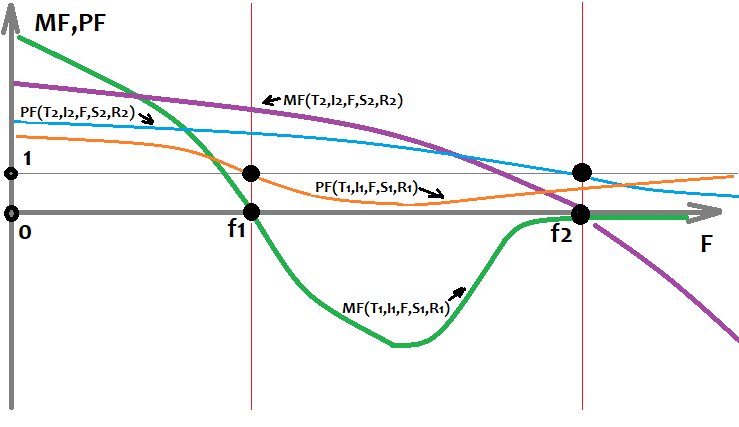### 下一步怎样做?

1. 添加分析固定服务器时间窗口的功能。
2. 添加在一周的固定日期执行分析的功能。
3. 添加随机生成时间窗口的功能。
4. 添加随机生成一周中允许交易的天数数组的功能。
5. 改进分析的第二阶段（优化选项卡），特别是多核计算的实现和优化器错误的纠正。

## 量化课程

移动端课程